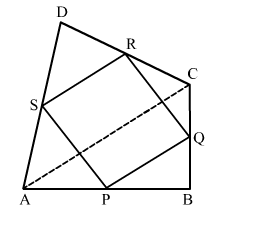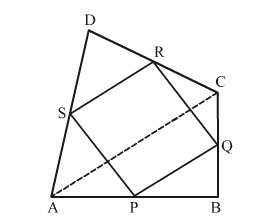# P, Q, R and S are respectively the midpoints of the sides AB, BC, CD and DA of a quadrilateral ABCD. Show that

Question:

P, Q, R and S are respectively the midpoints of the sides ABBCCD and DA of a quadrilateral ABCD. Show that

(i) $P Q \| A C$ and $P Q=\frac{1}{2} A C$

(ii) PQ || SR

(iii) PQRS is a parallelogram.Solution:Given: In quadrilateral ABCD, P, Q, R and S are respectively the midpoints of the sides AB, BC, CD and DA.

To prove:

(i) $P Q \| A C$ and $P Q=\frac{1}{2} A C$

(ii) $P Q \| S R$

(iii) $P Q R S$ is a parallelogram

Proof:

(i)

In $\Delta A B C$

Since, P and Q are the mid points of sides AB and BC, respectively.      (Given)

$\Rightarrow A C \| P Q$ and $P Q=\frac{1}{2} A C$       (Using mid-point theorem.)

(ii)

In $\triangle A D C$,

Since, S and R are the mid-points of AD and DC, respectively.        (Given)

$\Rightarrow S R \| A C$ and $S R=\frac{1}{2} A C$               (Using mid-point theorem.)            ...(1)

From (i) and (1), we get

PQ || SR

(iii)
From (i) and (ii), we get

$P Q=S R=\frac{1}{2} A C$

So, PQ and SR are parallel and equal.

Hence, PQRS is a parallelogram.